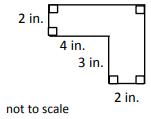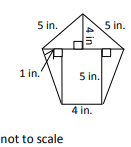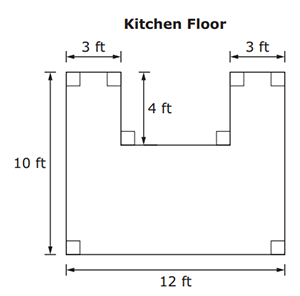# MAFS.6.G.1.1Archived StandardExport Print
Find the area of right triangles, other triangles, special quadrilaterals, and polygons by composing into rectangles or decomposing into triangles and other shapes; apply these techniques in the context of solving real-world and mathematical problems.
General Information
Subject Area: Mathematics
Domain-Subdomain: Geometry
Cluster: Level 2: Basic Application of Skills & Concepts
Cluster: Solve real-world and mathematical problems involving area, surface area, and volume. (Supporting Cluster) -

Clusters should not be sorted from Major to Supporting and then taught in that order. To do so would strip the coherence of the mathematical ideas and miss the opportunity to enhance the major work of the grade with the supporting clusters.

Date of Last Rating: 02/14
Status: State Board Approved - Archived
Assessed: Yes
Test Item Specifications

• Assessment Limits :
Numbers in items must be nonnegative rational numbers. Limit shapes to those that can be decomposed or composed into rectangles and/or right triangles.
• Calculator :

No

• Context :

Allowable

Sample Test Items (3)
• Test Item #: Sample Item 1
• Question:

A shape is shown.What is the area, in square inches, of the shape?

• Difficulty: N/A
• Type: EE: Equation Editor

• Test Item #: Sample Item 2
• Question:

A pentagon is shown.What is the area, in square inches, of the pentagon?

• Difficulty: N/A
• Type: EE: Equation Editor

• Test Item #: Sample Item 3
• Question:

Mr. Hilton is buying new tile for his kitchen floor. The dimensions, in feet (ft), of the kitchen floor are shown.What is the area, in square feet, of Mr. Hilton's kitchen floor?

• Difficulty: N/A
• Type: EE: Equation Editor

## Related Courses

This benchmark is part of these courses.
1205010: M/J Grade 6 Mathematics (Specifically in versions: 2014 - 2015, 2015 - 2022, 2022 and beyond (current))
1205020: M/J Accelerated Mathematics Grade 6 (Specifically in versions: 2014 - 2015, 2015 - 2020, 2020 - 2022, 2022 and beyond (current))
1204000: M/J Foundational Skills in Mathematics 6-8 (Specifically in versions: 2014 - 2015, 2015 - 2022, 2022 and beyond (current))
0101010: M/J Two-Dimensional Studio Art 1 (Specifically in versions: 2014 - 2015, 2015 - 2022, 2022 and beyond (current))
0101020: M/J Two-Dimensional Studio Art 2 (Specifically in versions: 2014 - 2015, 2015 - 2022, 2022 and beyond (current))
0101040: M/J Three-Dimensional Studio Art 1 (Specifically in versions: 2014 - 2015, 2015 - 2022, 2022 and beyond (current))
0101050: M/J Three-Dimensional Studio Art 2 (Specifically in versions: 2014 - 2015, 2015 - 2022, 2022 and beyond (current))
7812015: Access M/J Grade 6 Mathematics (Specifically in versions: 2014 - 2015, 2015 - 2018, 2018 - 2022, 2022 and beyond (current))
0101025: M/J Two-Dimensional Studio Art 2 & Career Planning (Specifically in versions: 2014 - 2015, 2015 - 2019, 2019 - 2022, 2022 and beyond (current))
0101005: M/J Exploring Two-Dimensional Art (Specifically in versions: 2014 - 2015, 2015 - 2022, 2022 and beyond (current))
0101026: M/J Two-Dimensional Studio Art 3 (Specifically in versions: 2014 - 2015, 2015 - 2022, 2022 and beyond (current))
0101035: M/J Exploring Three-Dimensional Art (Specifically in versions: 2014 - 2015, 2015 - 2022, 2022 and beyond (current))
0101060: M/J Three-Dimensional Studio Art 3 (Specifically in versions: 2014 - 2015, 2015 - 2022, 2022 and beyond (current))
7912110: Fundamental Explorations in Mathematics 1 (Specifically in versions: 2013 - 2015, 2015 - 2017 (course terminated))

## Related Access Points

Alternate version of this benchmark for students with significant cognitive disabilities.

## Related Resources

Vetted resources educators can use to teach the concepts and skills in this benchmark.

## Formative Assessments

Swimming Pool Walkway:

Students are asked to solve a problem involving finding the area of a composite plane figure.

Type: Formative Assessment

Lost Key:

Students are asked to find the area of a composite plane figure.

Type: Formative Assessment

Students are asked to find the area of a trapezoid and a parallelogram by composing or decomposing into triangles and rectangles.

Type: Formative Assessment

Area of Kite:

Students are asked to find the area of a kite by composing it into rectangles or decomposing it into triangles.

Type: Formative Assessment

Area of Triangles:

Students are asked to find the area of two different triangles.

Type: Formative Assessment

## Lesson Plans

Coding Geometry Challenge 8, 9 & 17:

This set of geometry challenges focuses on using area/perimeter as students problem solve and think as they learn to code using block coding software.  Student will need to use their knowledge of the attributes of polygons and mathematical principals of geometry to accomplish the given challenges. The challenges start out fairly simple and move to more complex situations in which students can explore at their own pace or work as a team. Computer Science standards are seamlessly intertwined with the math standards while providing “Step it up!” and “Jump it up!” opportunities to increase rigor.

Type: Lesson Plan

Coding Geometry Challenge # 16, 18 & 19:

This set of geometry challenges focuses on creating a variety of polygons using the coordinate plane as students problem solve and think as they learn to code using block coding software.  Student will need to use their knowledge of the attributes of polygons and mathematical principals of geometry to accomplish the given challenges. The challenges start out fairly simple and move to more complex situations in which students can explore at their own pace or work as a team. Computer Science standards are seamlessly intertwined with the math standards while providing “Step it up!” and “Jump it up!” opportunities to increase rigor.

Type: Lesson Plan

Dream House Project :

Students will design a floor plan of their dream house using compositions of basic geometric shapes. They will also calculate the area of the plan to determine flooring costs. Students will conduct research on alternative energy sources and determine the best fit for their dream house location.

Type: Lesson Plan

Breaking Up is Hard to Do:

Student will use geoboards to decompose composite figures and polygons into squares, rectangles, and triangles in order to find the total area.

Type: Lesson Plan

Blown Away:

A STEM Engineering Design Challenge

Learning Goals

• Students will be able to demonstrate an understanding of hazardous weather conditions, specifically hurricanes, and identify ways for humans to protect themselves during those conditions.
• Students will understand how area of various shapes can enhance the stability of a structure.

Students will create a free-standing structure that can withstand hurricane-force winds. Students will demonstrate their understanding of surface area by constraining to sets of parameters for their structures.

Type: Lesson Plan

Using Security Camera Angles to Find Area and Calculate Percentages:

In this lesson, students work individually and then collectively using a real world situation to construct sight lines to see which areas are visible from a security camera. Students then find and compare the area of triangles and quadrilaterals and compare and calculate the percentages and/or fractions of areas.

Type: Lesson Plan

Area of a Triangle:

This lesson is primarily formative in nature and is designed to introduce students to the area of a triangle by having them derive the formula themselves using the relationship between rectangles and triangles. During the lesson the teacher will be facilitating their students as they work with their teams and shoulder partners to solve problems.

Type: Lesson Plan

Building a Tree House:

This MEA will have students determining the safest and most cost effective material to use when building a tree house.They will do this by calculating surface area and determining cost.

Type: Lesson Plan

Profit Plaza:

This lesson requires students to use mathematical data and logic/reasoning to place vendors into retail spaces in a shopping plaza. Students will first rank five vendor types on their profitability (based on average sales and average overhead/upkeep costs), then place the vendor types into the 11-13 retail spaces. They are also required to find the area of each space and calculate the total leasing charges. The plans for the plaza are given on a coordinate plane, so students will need to find the lengths of horizontal and vertical line segments (using the coordinates of the endpoints) to calculate the areas of the rectangular and composite spaces.

Type: Lesson Plan

Finding Area with Hands-On Measurement:

This lesson allows students to apply the area of triangles, quadrilaterals, and trapezoids to composite figures, and gives students a chance to work with classmates to find the area by taking measurements and making the necessary calculations. Students will also see the relationship between the area formulas for rectangles, triangles, trapezoids, and polygons.

Type: Lesson Plan

Area of a Right Triangle:

Area of a Right Triangle

Type: Lesson Plan

Enrique's Ruined Carpet:

In this activity, students use a house blueprint to find the area of carpeted floor by decomposing composite shapes into rectangles and triangles. As students critique each other's reasoning, they refine their thinking of surface area.

Type: Lesson Plan

## Original Student Tutorials

Area of Triangles:

Follow George as he explores the formula for the area of a triangle and uses it to find the area of various triangles in this interactive student tutorial.

Type: Original Student Tutorial

Area of Triangles: Missing Dimensions:

Follow George as he calculates the missing values for the base and height of triangles in this interactive tutorial.

Type: Original Student Tutorial

Maximizing Area: Gold Rush:

Before the lesson, students attempt the Gold Rush task individually. You then look at their responses and formulate questions for students to think about as they review their work. At the start of the lesson, students reflect on their individual responses and use the questions posed to think of ways to improve their work. Next, students work collaboratively in small groups to produce, in the form of a poster, a better solution to the Gold Rush task than they did individually. In a whole-class discussion students compare and evaluate the different methods they used. Working in small groups, students analyze sample responses to the Gold Rush task, then, in a whole-class discussion, review the methods they have seen. Finally, students reflect on their work.

Base and Height:

Students are asked to determine and illustrate all possible descriptions for the base and height of a given triangle.

Finding Areas of Polygons, Variation 1:

Students are asked to demonstrate two different strategies for finding the area of polygons shown on grids.

Painting a Barn:

Students are asked to use the given information to determine the cost of painting a barn.

Same Base and Height, Variation 1:

This task is a good precursor to students developing the formula for the area of a triangle. The fact that each triangle has the same area can be used to highlight the meaning of the components of the area formula, as well as the meaning of the altitude of a triangle (an issue since the given triangles are not acute.) Students may try to determine the area of each triangle by counting the square units or using the "surround and subtract" method. Students may think that triangle ABC has the largest area because the others appear thinner.

Same Base and Height, Variation 2:

This is the second version of a task asking students to find the areas of triangles that have the same base and height. This presentation is more abstract as students are not using physical models. They still determine the area of each triangle by counting the square units or using the "surround and subtract" method, but it is a good lead-up for students to think about the formula for the area of a triangle and notice that the length of bases and altitudes are the same. Students who do not analyze the area may think that triangle ABC has the largest area because the others appear thinner.

## Student Center Activity

Students can practice answering mathematics questions on a variety of topics. With an account, students can save their work and send it to their teacher when complete.

Type: Student Center Activity

## Tutorials

Finding Area by Decomposing a Shape:

This tutorial demonstrates how the area of an irregular geometric shape may be determined by decomposition to smaller familiar shapes.

Type: Tutorial

Area of a Parallelogram:

This video portrays a proof of the formula for area of a parallelogram.

Type: Tutorial

Area of a Trapezoid:

A trapezoid is a type of quadrilateral with one set of parallel sides. Here we explain how to find its area.

Type: Tutorial

Area of Triangle on a Grid:

We will be able to find the area of a triangle in a coordinate grid. The formula for the area of a triangle is given in this tutorial.

Type: Tutorial

Perimeter and Area:

Students will learn the basics of finding the perimeter and area of squares and rectangles.

Type: Tutorial

## Virtual Manipulatives

Area Builder:

This manipulative allows you to create shapes using colorful blocks to explore the relationship between perimeter and area. The game screen challenges you to build shapes or find the area of figures.

Type: Virtual Manipulative

Triangle Area:

This lesson is designed to introduce students to calculating the area of right triangles. This lesson provides links to discussions and activities related to area as well as suggested ways to integrate them into the lesson. Finally, the lesson provides links to follow-up lessons designed for use in succession with the current one.

Type: Virtual Manipulative

## STEM Lessons - Model Eliciting Activity

Building a Tree House:

This MEA will have students determining the safest and most cost effective material to use when building a tree house.They will do this by calculating surface area and determining cost.

Profit Plaza:

This lesson requires students to use mathematical data and logic/reasoning to place vendors into retail spaces in a shopping plaza. Students will first rank five vendor types on their profitability (based on average sales and average overhead/upkeep costs), then place the vendor types into the 11-13 retail spaces. They are also required to find the area of each space and calculate the total leasing charges. The plans for the plaza are given on a coordinate plane, so students will need to find the lengths of horizontal and vertical line segments (using the coordinates of the endpoints) to calculate the areas of the rectangular and composite spaces.

## MFAS Formative Assessments

Area of Kite:

Students are asked to find the area of a kite by composing it into rectangles or decomposing it into triangles.

Students are asked to find the area of a trapezoid and a parallelogram by composing or decomposing into triangles and rectangles.

Area of Triangles:

Students are asked to find the area of two different triangles.

Lost Key:

Students are asked to find the area of a composite plane figure.

Swimming Pool Walkway:

Students are asked to solve a problem involving finding the area of a composite plane figure.

## Original Student Tutorials Mathematics - Grades 6-8

Area of Triangles:

Follow George as he explores the formula for the area of a triangle and uses it to find the area of various triangles in this interactive student tutorial.

Area of Triangles: Missing Dimensions:

Follow George as he calculates the missing values for the base and height of triangles in this interactive tutorial.

## Student Resources

Vetted resources students can use to learn the concepts and skills in this benchmark.

## Original Student Tutorials

Area of Triangles:

Follow George as he explores the formula for the area of a triangle and uses it to find the area of various triangles in this interactive student tutorial.

Type: Original Student Tutorial

Area of Triangles: Missing Dimensions:

Follow George as he calculates the missing values for the base and height of triangles in this interactive tutorial.

Type: Original Student Tutorial

Base and Height:

Students are asked to determine and illustrate all possible descriptions for the base and height of a given triangle.

Finding Areas of Polygons, Variation 1:

Students are asked to demonstrate two different strategies for finding the area of polygons shown on grids.

Painting a Barn:

Students are asked to use the given information to determine the cost of painting a barn.

## Student Center Activity

Students can practice answering mathematics questions on a variety of topics. With an account, students can save their work and send it to their teacher when complete.

Type: Student Center Activity

## Tutorials

Finding Area by Decomposing a Shape:

This tutorial demonstrates how the area of an irregular geometric shape may be determined by decomposition to smaller familiar shapes.

Type: Tutorial

Area of a Parallelogram:

This video portrays a proof of the formula for area of a parallelogram.

Type: Tutorial

Area of a Trapezoid:

A trapezoid is a type of quadrilateral with one set of parallel sides. Here we explain how to find its area.

Type: Tutorial

Area of Triangle on a Grid:

We will be able to find the area of a triangle in a coordinate grid. The formula for the area of a triangle is given in this tutorial.

Type: Tutorial

Perimeter and Area:

Students will learn the basics of finding the perimeter and area of squares and rectangles.

Type: Tutorial

## Parent Resources

Vetted resources caregivers can use to help students learn the concepts and skills in this benchmark.

Base and Height:

Students are asked to determine and illustrate all possible descriptions for the base and height of a given triangle.